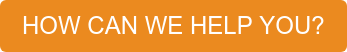##### Resources / Articles / Mechanical Resonant Frequency and How to Analyze It# Mechanical Resonant Frequency and How to Analyze It

At one point in their career, a system engineer will probably run into a case where the mechanical resonant frequency of a system is causing motion control problems and/or an audible annoyance. This electrical resonance, or vibration, is not desirable and will lead to significant performance degradation. For example, a CNC milling machine (which typically has a high resonant frequency due to high stiffness)  will jitter around the desired milling path if the resonant frequency is excited. Another possible occurrence would be a pharmaceutical discovery instrument with unexpected vibrations that will agitate the liquid solution it’s transporting. This article will explore why the resonant frequency is present and what tools can be used to analyze it.

## Why do mechanical systems exhibit a resonant frequency?

The first step in answering this question is to create a model of a mechanical system. Determining the position of a mass, with frictional and compliance terms included, requires that the model be a system of one or more 2nd order (or higher) differential equation(s). To find the solution, as well as ease analysis of behavior, the unsolved time domain equations are transformed into the s-domain by using a Laplace transform. This facilitates the creation of a system level transfer function which is also referred to as a “characteristic equation”. The denominator of the transfer function contains the state variable (s) of order n. The values of s (real and/or imaginary) where the denominator equals zero are referred to as roots of the denominator which are called “poles”. The numerator may be a constant or it may contain the state variable (s) of order n, similar to the denominator. The values of s (real and/or imaginary) where the numerator equals zero are referred to as roots of the numerator which are called “zeros”. If the numerator is a constant then there are no zeros. This information can be used to formulate a time domain solution for the model. However, the frequency analysis used here will be limited to the s-domain for ease of computation.

The poles and zeros are very useful for determining behavior and stability. For example, stability criteria includes that the order of the denominator (number of poles) must not be less than the order of the numerator (number of zeros); also the real part of the dominant poles must be negative (left-hand side of the s-plane) in order for the system to be considered stable. The poles will be used here to explain the existence of the resonant frequency.

Inertial systems have a resonant frequency which exists as a result of inherent properties. However, the system needs to be more complex than purely rigid body inertia otherwise its characteristic equation will have only poles at zero. Other terms in the characteristic equation, which can be as simple as a compliance term (spring), will introduce non-zero frequency components (roots with imaginary parts) in the characteristic equation. Purely inertial systems will have poles at zero and therefore not exhibit a resonant frequency.

Since the “rigid body” is really just an academic construct that does not exist in the real world, even simple inertial systems do possess the necessary complexity to exhibit a mechanical resonant frequency when spring elements (compliance) and/or dampening components (viscous friction) are added to the model. Some systems are sufficiently dampened to suppress the resonant frequency. In this case the resonance still exists (the imaginary part of the roots) but is barely noticeable since the real parts of the roots dominate the response.

## Demonstration Setup

The system being used in this demonstration (Figure 1) is a mass at the top of a metal ruler which will act like a spring. The bottom of the spring is attached to the stage of a linear motor. For simplicity, only motion in the direction of the linear motor will be analyzed. The motion of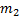will be treated as linear which is a valid approximation for small angles. This simplification is still valid in the context of describing the source of the resonant frequency. Also, only the position of the motor,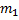, is measured. The model contains a system dampening coefficient,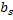, which imparts a force proportional to the velocity and opposite the direction of motion. This property is commonly referred to as “viscous friction” and represents any friction-like properties that are proportional to velocity control. The force being applied to the system by the motor’s magnetic field is controlled by manipulating the current in the motor windings.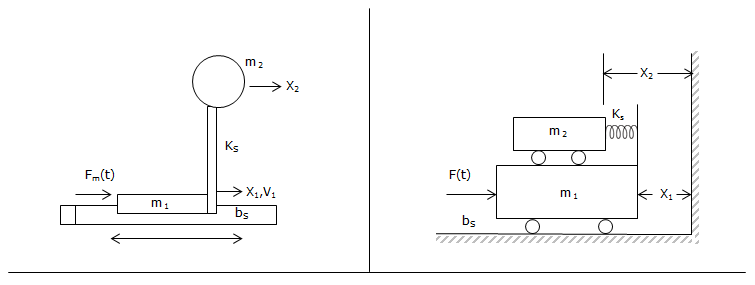Figure 1 – Demonstration System

The position response ofto a force exerted on this system is expressed with a resonant frequency calculation: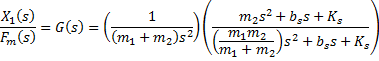(eq. 1) -Derived from torsional velocity response in Reference 1

Note that the term on the left of the right hand side of the equation is the “non-coupled” response. If the spring is of infinite stiffness the term on the far right equals one and the two mass are rigidly attached and act as one mass. In this case there are only poles at zero and therefore no resonance.

The term on the right introduces roots with imaginary components, thus creating the potential for a frequency response. For the case whereis zero the roots of this term become: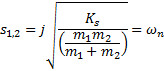(eq. 2)

This is also referred to as the “undamped natural frequency” of the system. In the case where the dampening termis small (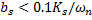) , the undamped natural frequency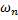is the resonant frequency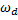.

The introduction of a moderate dampening term (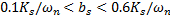)  will introduce a real component to the roots and move them such that the resonant frequency is slightly less than the undamped natural frequency. A stronger dampening term (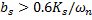)  will decrease the frequency even more but the response at the frequency will be attenuated and have little effect. In this case the real parts of the roots are dominant.

Because of the zero in the numerator this model will also exhibit an “anti-resonance” frequency. This can be observed as a decrease in the response at a given anti-resonance. Coupled-mass systems like the one being analyzed here will contain an anti-resonance frequency just below the resonant frequency. Beyond the resonance, the response is larger than that of a system where the masses are rigidly coupled. The latter is where the vibration creates the most problems .

## Experimental Determination of Resonant Frequency

Using the resonant frequency definition as, “the frequency at which a system will exhibit a localized maximum response” means that an experiment can be run on the system where the response as a function of frequency is measured.

One tool, referred to as a frequency sweep, will inject a variable frequency signal into a system. The frequency input begins at a pre-defined value and changes in a continuous fashion until a defined maximum value is reached. Most mechanical systems have resonant frequencies in the hundreds of hertz or lower. If the resonance is significant it will usually produce an audible sound and a rough estimate can be made by the listener.

A similar tool, the Bode plot, will analyze the gain and phase of the response and generate a plot in the frequency domain. To simplify the calculation on a digital processor, a set of discrete frequencies are sampled and the results are run through an FFT (Fast Fourier Transform).

The following videos demonstrate the use of various tools in Pro-Motion to determine the resonant frequency of the system in Figure 1.

Method #1: Calculation based on Actual Position trace of disturbance response.

A disturbance is injected into the system. The Pro-Motion SCOPE feature is used to track the response of the position of. The mechanical resonant frequency is the inverse of the period of time between one peak to the next. From the video it can be observed that the period is pretty close to 100ms. This corresponds to a resonant frequency of 10Hz.

Method #2: A Frequency Sweep is used to sweep from a low to high frequency.

A sinusoidal force is exerted on the system (via the magnetic field of the motor). The oscillation of the system is visually (or audibly) observed. The frequency of the local maximum response is noted. From Method #1 the approximate resonant frequency is already known. However, if the system settles quickly in response to the injected “pulse” of Method #1 than there may not be enough data to determine the resonant frequency. The continuous disturbance injected by Method #2 will provide more data in that regards since the system never settles. As can be seen in the video, the amplitude of the oscillations of the actual position begins to increase around the 5.2 second mark (11Hz). The frequency continues to increase to 14Hz and the response dies out after it passes the resonant frequency. The sweep then reverses direction and moves from 14Hz down to 10.5 Hz and remains there. As expected the amplitude of the response increases as the resonant frequency is approached.

Method #3: A Bode plot of the system is created covering the same range of frequencies.

Once again a sinusoidal force is exerted on the system. This time the position response in relation to the injected force is used to calculate the gain and phase of the response. In this case the user does not need to rely on visual or audible clues. The user can analyze the gain data to find the local maximum and the frequency associated with that maximum. For a more detailed analysis the numeric data can be ported into a spreadsheet and analyzed.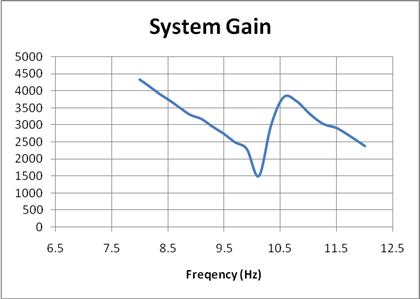Figure 2: Bode gain data plotted in a spreadsheet.

Figure 2 demonstrates a more precise determination of the resonant frequency (10.6 Hz). The anti-resonant frequency can also be observed in Figure 2 at about 10.1 Hz.

## At the end of the day…

Because no inertial system contains true “rigid body” masses, all mechanical systems exhibit a non-zero resonance. Excitation of the resonant frequency will degrade system performance in both short term accuracy and long term maintenance issues.

Three methods were demonstrated for experimental determination of the resonant frequency. Method #1 provided a result within 10% but this method will be limited to systems that have a under damped response to an impulse. Method #2, which relies on visual and audio clues, yields an equivalent accuracy and will work on both over damped and under damped systems. Method #3 provided the most detail about the system frequency response. The accuracy of the resonant frequency estimation was improved significantly. Additionally, this method also allowed for the identification of the anti-resonant frequency.

### References:

1 G. Ellis, Cures for Mechanical Resonance in Industrial Servo Systems
2 J. D’Azzo and C. Houpis, Linear Control System Analysis and Design: Conventional and Modern,  McGraw-Hill, Inc, 1995, pgs. 292-293.

## You may also be interested in: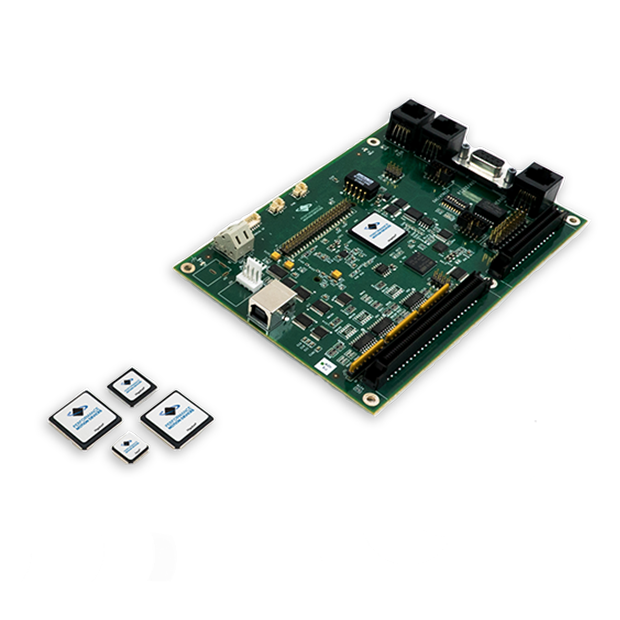### Stay ahead of the competition.

If your existing equipment needs a performance boost or if you need to develop a new product in the shortest development time possible, learn about motion control solutions from Performance Motion Devices.

• 2X faster development
• Motion solutions from ICs to turn-key boards
• Easy to implement
• Always supported